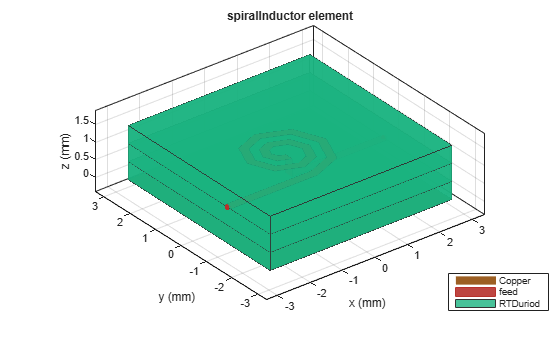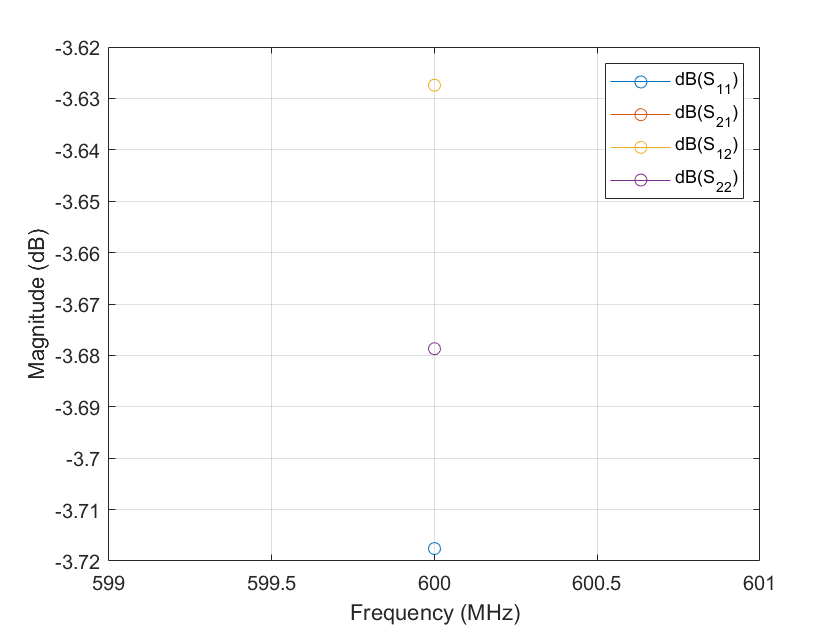# spiralInductor

Create spiral inductor in four different shapes

Since R2021b

## Description

Use the `spiralInductor` object to create a spiral inductor in one of four different shapes: square, circle, hexagon, or octagon. The spiral inductor is a two-port planar inductor with a single or multiple dielectric layers. A turn in a spiral inductor is the length of a complete 360-degree revolution. Spiral inductor filaments have uniform spacing and width throughout the structure. Spiral inductors are an integral part of many radio-frequency and microwave circuits, acting as resonant elements or chokes. The inductor feed can be configured in one of the following two ways:

Note

This PCB object supports behavioral modeling. For more information, see Behavioral Models. To analyze the behavioral model for a spiral inductor, set the `Behavioral` property in the `sparameters` function to `true or 1`

• The input and output ports are punched through at the same layer.

• The input port is routed out from the layer below the inductor by a via hole. The output port is extended to the end of the dielectric in the same layer.## Creation

### Syntax

``inductor = spiralInductor``
``inductor = spiralInductor(Name=Value)``

### Description

example

````inductor = spiralInductor` creates a square spiral planar inductor with default properties for a resonant frequency of 600 MHz.```

example

````inductor = spiralInductor(Name=Value)` sets Properties using one or more name-value arguments. For example, `spiralInductor(SpiralShape="Octagon")` creates an octagonal spiral inductor. Properties not specified retain their default values.```

## Properties

expand all

Shape of the spiral inductor, specified as either `"Square"`, `"Circle"`, `"Hexagon"`, or `"Octagon"`.

Example: `inductor = spiralInductor(SpiralShape="Circle")`

Data Types: `string` | `char`

Inner diameter of the polygon along the edge in meters, specified as a positive scalar.

Example: `inductor = spiralInductor(InnerDiameter=8.0000e-04)`

Data Types: `double`

Strip width in meters, specified as a positive scalar.

Example: `inductor = spiralInductor(Width=3.8000e-04)`

Data Types: `double`

Distance between the strips in meters, specified as a positive scalar.

Example: `inductor = spiralInductor(Spacing=3.8000e-04)`

Data Types: `double`

Number of turns in the spiral inductor, specified as a positive scalar. You can specify a minimum of `1` turn and a maximum of `12` turns. One turn length is the length of a complete 360-degree revolution.

Example: `inductor = spiralInductor(NumTurns=6)`

Data Types: `double`

Height from the ground plane to the inductor in meters, specified as a positive scalar.

Example: `inductor = spiralInductor(Height=0.0056)`

Data Types: `double`

Length of the ground plane in meters, specified as a positive scalar. This object does not support infinite ground plane length.

Example: `inductor = spiralInductor(GroundPlaneLength=0.046)`

Example: double

Width of the ground plane in meters, specified as a positive scalar. This object does not support infinite ground plane width.

Example: `inductor = spiralInductor(GroundPlaneWidth=0.046)`

Example: double

Type of dielectric material used as a substrate, specified as a `dielectric` object. The dielectric material in a `spiralInductor` object with default properties have the following properties:

• `Name``{'RTDuroid','RTDuroid','RTDuroid'}`

• `EpsilonR``[3.66,3.66,3.66]`

• `LossTangent``[0.0013,0.0013,0.0013]`

• `Thickness``[0.508e-3,0.508e-3,0.508e-3]`

Example: `d = dielectric("FR4");` `inductor = spiralInductor(Substrate=d)`

Data Types: `string` | `char`

Type of metal used in the conducting layers, specified as a `metal` object. The type of metal in a `spiralInductor` object with default properties is Copper

Example: `m = metal("PEC");` `inductor = spiralInductor(Conductor=m)`

Data Types: `string` | `char`

## Object Functions

 `charge` Calculate and plot charge distribution `current` Calculate and plot current distribution `feedCurrent` Calculate current at feed port `getZ0` Calculate characteristic impedance of transmission line `inductance` Calculate inductance `layout` Plot all metal layers and board shape `mesh` Change and view mesh properties of metal or dielectric in PCB component `shapes` Extract all metal layer shapes of PCB component `show` Display PCB component structure or PCB shape `sparameters` Calculate S-parameters for RF PCB objects

## Examples

collapse all

Create and view a default spiral inductor.

`inductor = spiralInductor`
```inductor = spiralInductor with properties: SpiralShape: 'Square' InnerDiameter: 5.0000e-04 Width: 2.5000e-04 Spacing: 2.5000e-04 NumTurns: 4 Height: 0.0010 GroundPlaneLength: 0.0056 GroundPlaneWidth: 0.0056 Substrate: [1x1 dielectric] Conductor: [1x1 metal] ```
`show(inductor)`Create and view a two-turn octagonal spiral inductor.

```inductor = spiralInductor(SpiralShape="Octagon",NumTurns=2); show(inductor)```Measure the inductance of the inductor.

```figure inductance(inductor,600e6)````ind = inductance(inductor,600e6)`
```ind = 1.0653e-08 ```

Create a spiral inductor using gold as the conductor.

```inductor = spiralInductor; inductor.Conductor = metal("Gold"); show(inductor)```Compute and plot the behavioral S-parameters of the inductor at 600 MHz.

```spar = sparameters(inductor,600e6,Behavioral=true); rfplot(spar)```